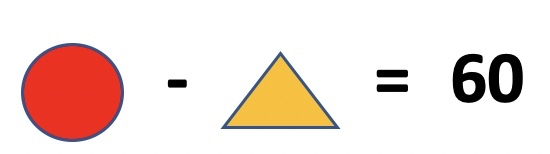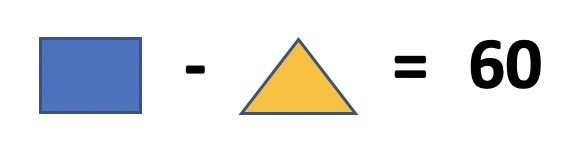# Olympiad Math Preparation – Class 2 – Computation Operations – Subtraction of 2 and 3 digit numbers ( without regrouping/borrow )

### Olympiad Math Preparation – Class 2 -Subtraction of 2 and 3 digit numbers ( without regrouping/borrow )

1. The difference between greatest and smallest number is _________________ . Numbers : 178, 608, 578, 801, 999

2. Select the correct option

3. The difference of numbers shown in two abacuses below is ________________

4. Ifandthen what is value of?

5. Ifis subtracted from 100, the result is 45. Thenis _________.

6. What is difference of largest and smallest 3 digit number ?

7. Duggu has 85 chocolates. Dakshu has 24 chocolates. How many more chocolates Duggu has than Dakshu ?

8.and. Find the value of